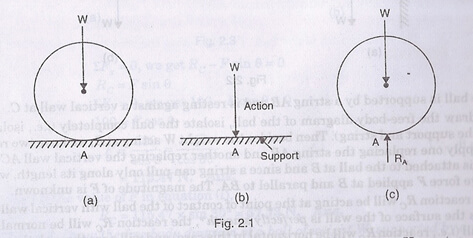In the equilibrium of bodies, if the supports are removed and replaced by the supportive reactions then the free body diagram can be understood. Consider the figure below:Here if the supportive surface is substituted with the reaction RA then that is an example of free body diagram.

• The point of application of the reaction RA will be the point of contact A, and from the law of equilibrium of two forces.
• We will concentrate upon that the reaction RA must vertical and equal to the weight W.
• Hence Fig.2.1 (c), in which the ball is completely isolated from its support and in which all forces acting on the ball are shown by vectors, is known a free-body diagram.

Link of Next Mechanical Engineering Topics:-

Free body diagram in equilibrium of rigid bodies### Customer Reviews

My Homework Help
Rated 5.0 out of 5 based on 510 customer reviews at